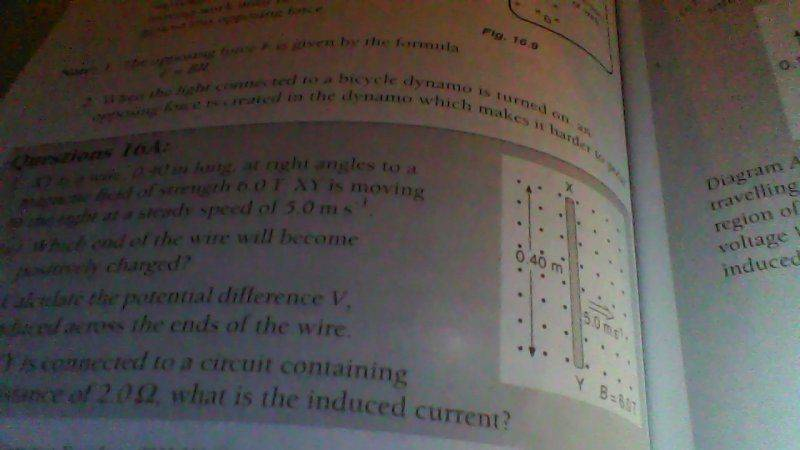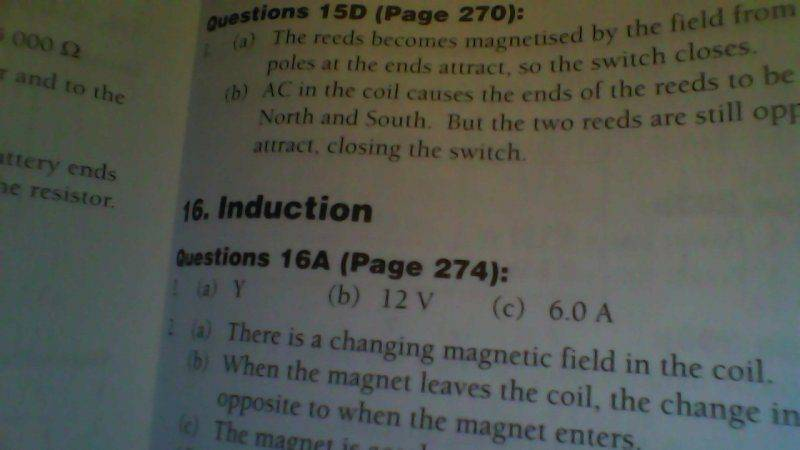# Textbook gives me a confusing answer to an induction question- want to know if it is wrong.

## Homework Statement

I have a question that involves a wire XY (X moving down the page to Y) moving to the right of the page at right angles to a magnetic field that acts out of the paper. The question then asks me which end of the wire will become positively charged. The textbook uses conventional current, and Fleming's right hand rule for induced currents.

2. The attempt at a solution
I know that current will move from X to Y as given by Fleming's right hand rule, and I know that conventional current is defined as being in the direction of positive charge flow (the opposite of the actual electron flow). By this logic, I assume that X will be positively charged. The textbook, however, states that Y will be positively charged.

Is the textbook wrong, or does Fleming's right hand rule give the direction of actual electron flow, rather than the direction of conventional current.

After some looking around, I can see that Fleming's Right-hand rule uses conventional current, but that doesn't explain to me why the textbook got the answer that it did.

ehild
Homework Helper
Instead of these hand-rules, you can use the expression of Lorentz force for moving charges in magnetic field. It is

## \vec F = q [ \vec v \times \vec B ]##, where q is the charge.

Set up a coordinate system. If the x axis points outward the page, and the wire moves in the y direction form left to right, the force will be parallel to the z axis (positive z is upward on the plane) . What is the direction of the force on the electrons, up or down? They will move to that end of the wire where the force points, so that end becomes negative.

Hi, According to your answer and the right hand rule related to it-The wikipedia page for Lorentz force says "positively charged particle... will curve perpendicularly to both the instantaneous velocity vector v and the B field according to the right-hand rule (in detail, if the thumb of the right hand points along v and the index finger along B, then the middle finger points along F)."- The force on the electron will downwards, thus Y should be negative, as I thought it would be. Could you just confirm this- I'll attach a picture of the diagram:Here is proof of the textbook answer:I just want to make sure that the textbook is wrong because I don't know if I'm just making the same mistake over and over again- even if you guys can't give me a straight answer for whatever reason, I'll probably ask my teacher because I just want to be 100% sure. I just thought I'd try here first, because sometimes my teacher gets confused himself.

ehild
Homework Helper
"in detail, if the thumb of the right hand points along v and the index finger along B, then the middle finger points along F" is valid for a positive charge. In that case, the force points downward, so Y becomes positive.

You may say that the positive ions in the metal can not migrate. Determine the Lorentz force exerted on the electrons: it is opposite, so it acts upward. The electrons accumulate at the top of the rod, X it becomes negative, Y positive.

If you use the cross product ##\vec F = q [ \vec v \times \vec B ]## with q= -e, ##\vec v = (0, V, 0)##, ##\vec B = (B, 0, 0)## ##\vec F = (0,0,eVB)##. An upward force acts on the electrons.

ehild

•Entanglement and karpmage
"in detail, if the thumb of the right hand points along v and the index finger along B, then the middle finger points along F" is valid for a positive charge. In that case, the force points downward, so Y becomes positive.
Oh I didn't see that the wikipedia article was refering to a positive charge. Thank you very much, I understand now why the electrons accumulate at Y. I'm going to use the Lorentz Force instead of Fleming's right hand rule for this kind of question. I also see now that the magnetic field has made electrons move from Y to X and thus the conventional current will be the opposite of this, from X to Y. Again, thank you so much, you have really helped me.

ehild
Homework Helper
You are welcome.

ehild

All of this confusion is because you think that the wire XY is the circuit,
So you say that since the electrons flow from Y to X then X must be positively charged and Y is negatively charged, and that's wrong.
Electrons were actually forced to move that way creating a potential difference,
So electrons are FORCED to move to X And they accumulate there under that FORCE so X becomes negatively charged and Y becomes positively charged, if you want a flowing current you must connect X and Y externally making a circuit, then, the electrons will flow from X to Y in the external wire.
You know the fact that electron naturally flow from negative to positive but it needs to be forced to move from
Plaice to negative. Basically, this is analogous to a battery and a circuit, XY is like a battery and the wire that was connected from X to Y externally is the circuit.
Electrons in a circuit flow from the negative pole to the positive pole naturally, but in the battery they are forced to do the opposite they flow from the positive pole to the negative pole.
Same thing with the XY wire, electrons flow naturally from X(negative pole) to Y (positive pole) externally but then they are forced to move from Y to X inside the wire XY.

Last edited: